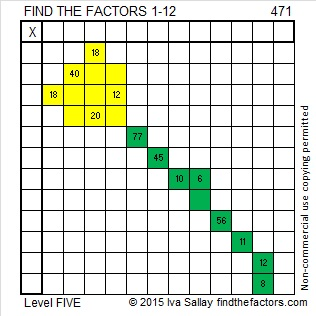# 471 and Level 5

471 is the sum of some consecutive prime numbers. One of my readers has listed those primes in the comments.Print the puzzles or type the solution on this excel file:  12 Factors 2015-04-20

—————————————————————————————————

• 471 is a composite number.
• Prime factorization: 471 = 3 x 157
• The exponents in the prime factorization are 1 and 1. Adding one to each and multiplying we get (1 + 1)(1 + 1) = 2 x 2 = 4. Therefore 471 has exactly 4 factors.
• Factors of 471: 1, 3, 157, 471
• Factor pairs: 471 = 1 x 471 or 3 x 471
• 471 has no square factors that allow its square root to be simplified. √471 ≈ 21.70253—————————————————————————————————